Astrophysical aspects of $r$-process nucleosynthesis in neutron star mergersLA-UR-19-24076

Matthew Mumpower

Notre Dame Astro. Seminar

Tuesday April 30$^{th}$ 2019Center for Theoretical
ASTROPHYSICS

Outline

Heavy element nucleosynthesis in mergers

Accretion disks

Morphology and composition

Future $\gamma$-ray observations

Concluding remarks

Neutron stars

Mass of around 1.1 to 3 M$_\odot$

Density $10^{9}$ to $10^{17}$ kg/m$^{3}$

Magnetic field $10^{10}$ Tesla

Color depends on equation of state...

Ozel & Freire ARA&A 54 401 (2016) • Figure by M. Mumpower

A close binary of neutron stars

GW170817 - named for the day it was discovered

NGC 4993 - about 40 Mpc away

Abbott et al. PRL 119 16 (2017) • Im et al. ApJL 849 1 (2017) • Figure by M. Mumpower

A close binary of neutron stars

Important for GCE

Coalescence time: ~1 million years

Cote et al. ApJ 855 2 (2018) • Figure by M. Mumpower

GW waves are emitted

Chirp mass $\sim 1.188$
implies NS binary

Abbott et al. PRL 119 16 (2017) • Smartt et al. Nature 551 75 (2017) • Figure by M. Mumpower

Neutron stars merge

Rosswog Nature 500 535 (2013) • Figure by M. Mumpower

Electromagnetic counterpart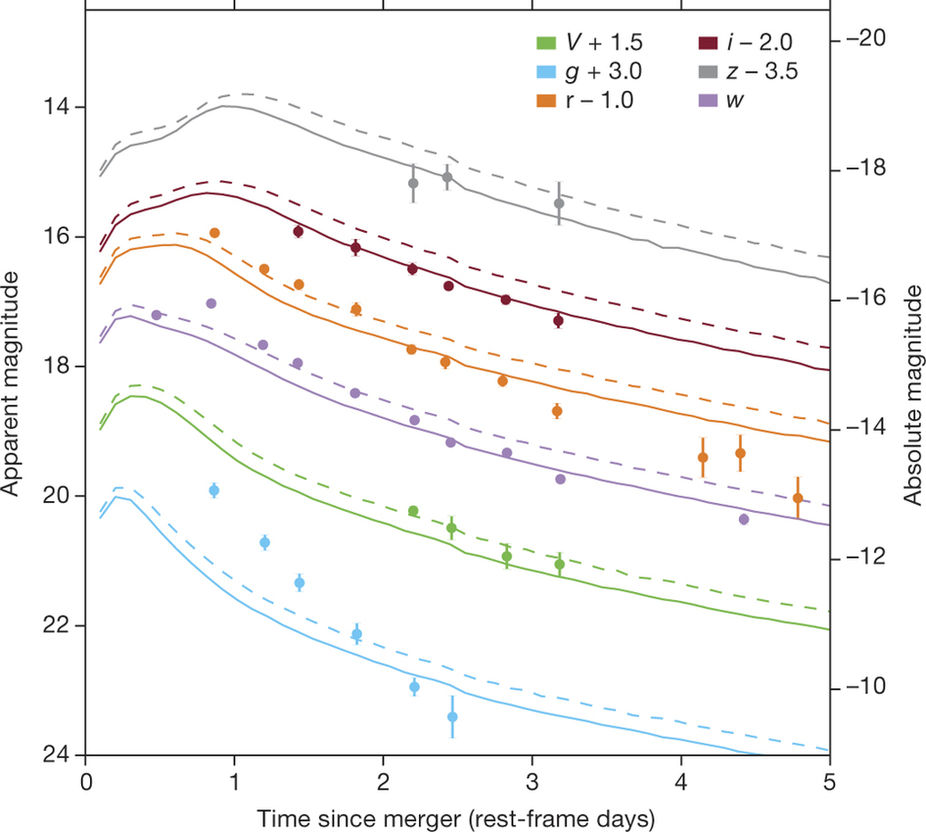Model assumptions: M$_{\textrm{ej}} = 10^{-2}$ M$_\odot$, V$_{\textrm{ej}}=0.3$c

Very low lanthanide fraction

Arcavi et al. Nature 551 64 (2017)

Electromagnetic counterpart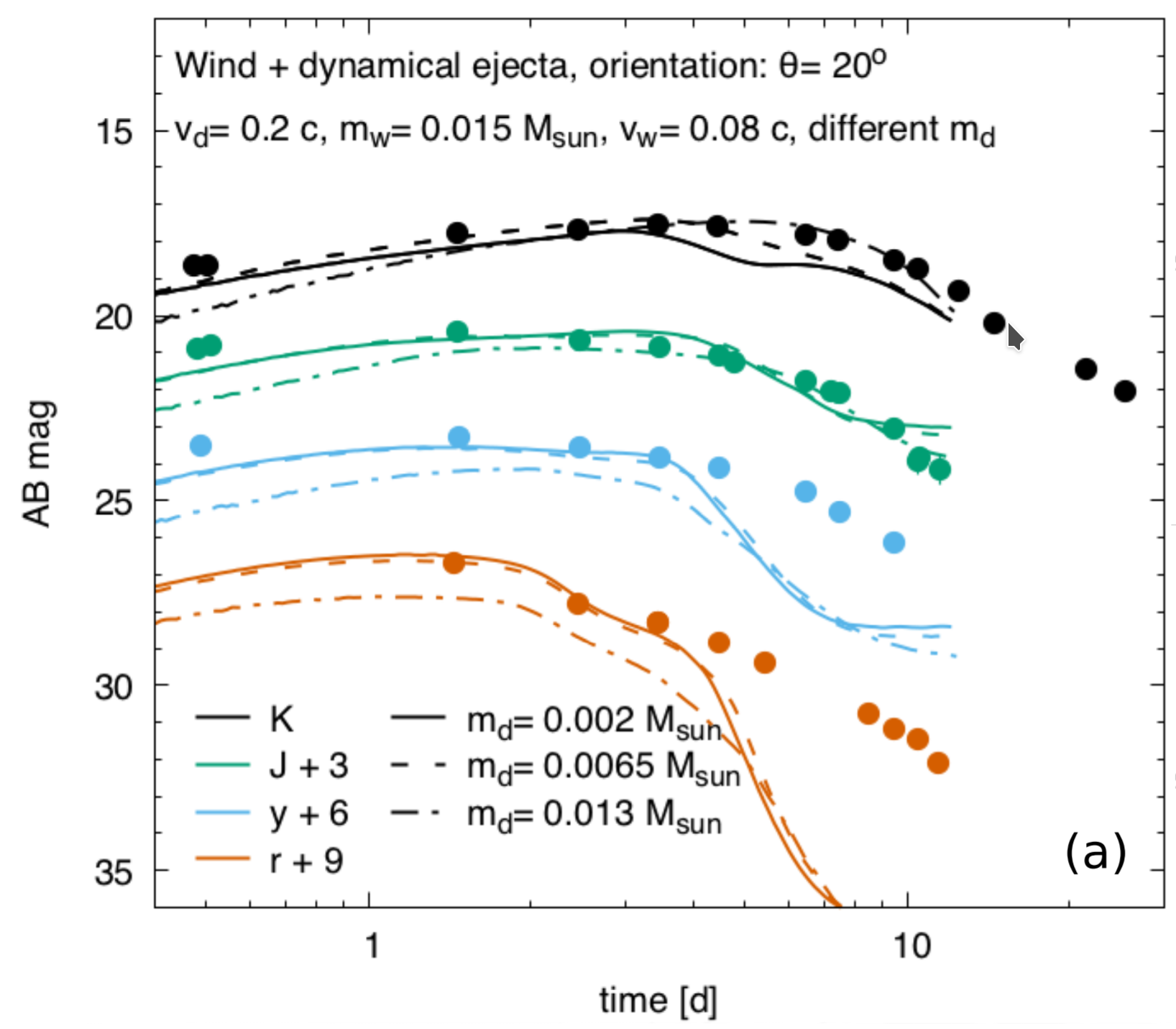To match data at later times

Some lanthanide production is needed

Tanvir et al. ApJL 848 2 (2017)

Schematic of a kilonova

2 component model: wind & dynamical eject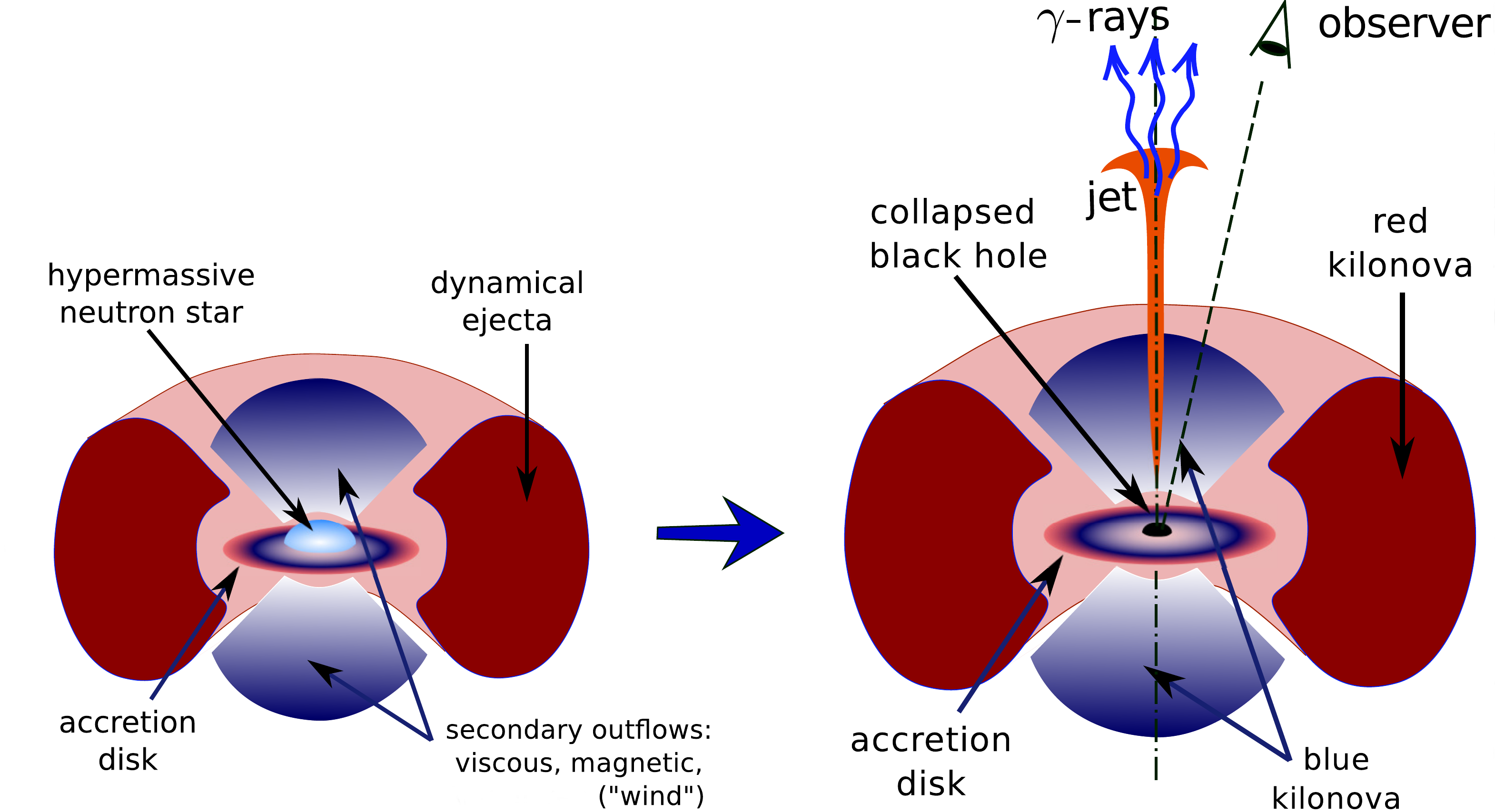Red emission: late (weeks); lanthanide dominated

blue emission: early (days); 1st 2nd peak dominated

Metzger et al. MNRAS 406 4 (2010) • Kasen et al. Nature 551 80 (2017) • Figure by O. Korobkin

Do mergers produce a solar like r-process?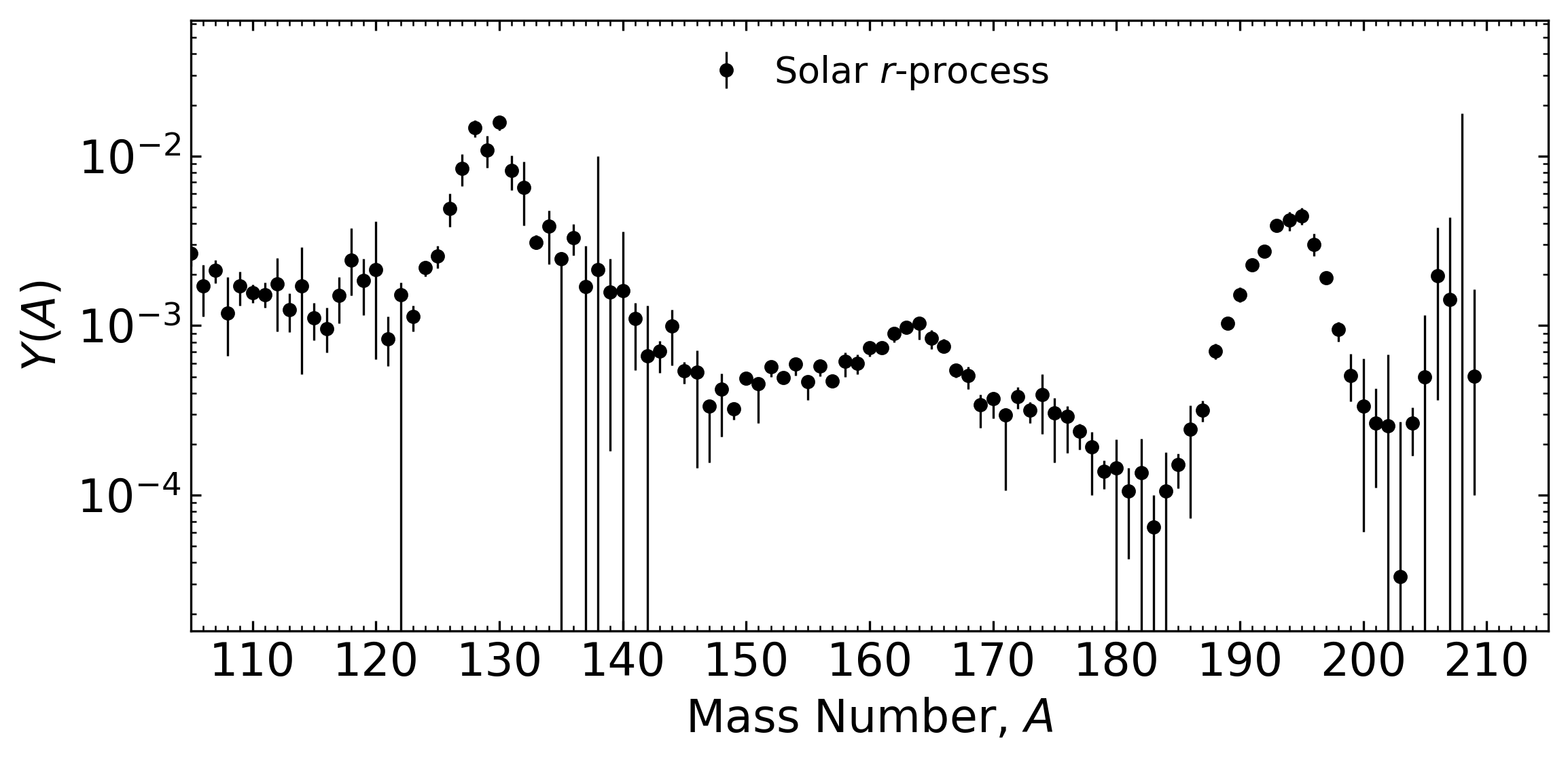To answer this question:

Knowledge of astrophysical conditions

Knowledge of nuclear physics inputs (1000's of unknown species / properties)

And precise observations!

Beers & Christlieb ARAA (2005) • Metzger et al. MNRAS (2010) • Barnes et al. ApJ (2016) • Côté et al. ApJ (2018)

Distribution of $Y_e$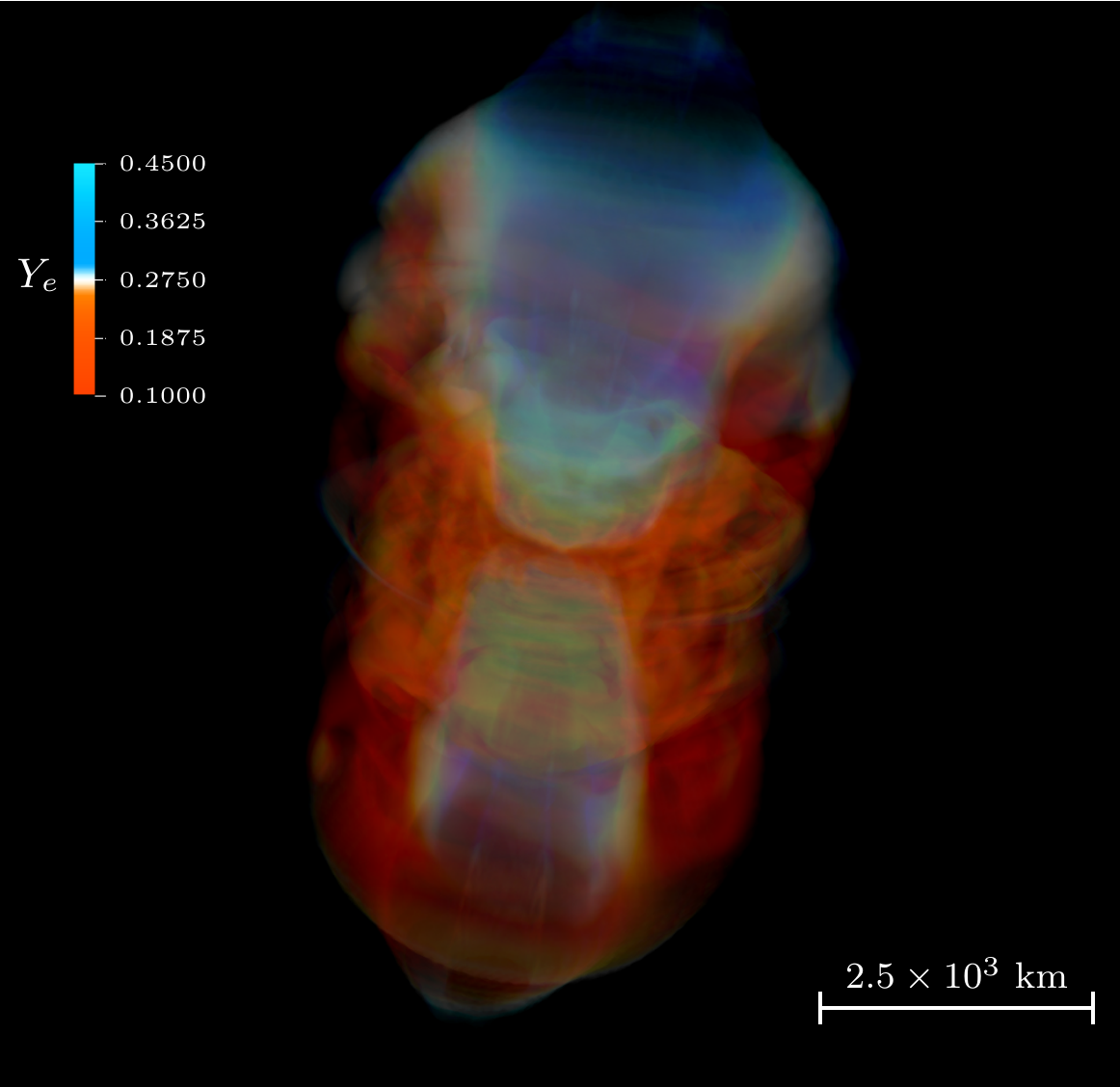Volume rendering of electron fraction, $Y_e$

Jet drives material and voids region near azimuthal axis; high $Y_e$

Miller et al. submitted (2019)

Accretion Disk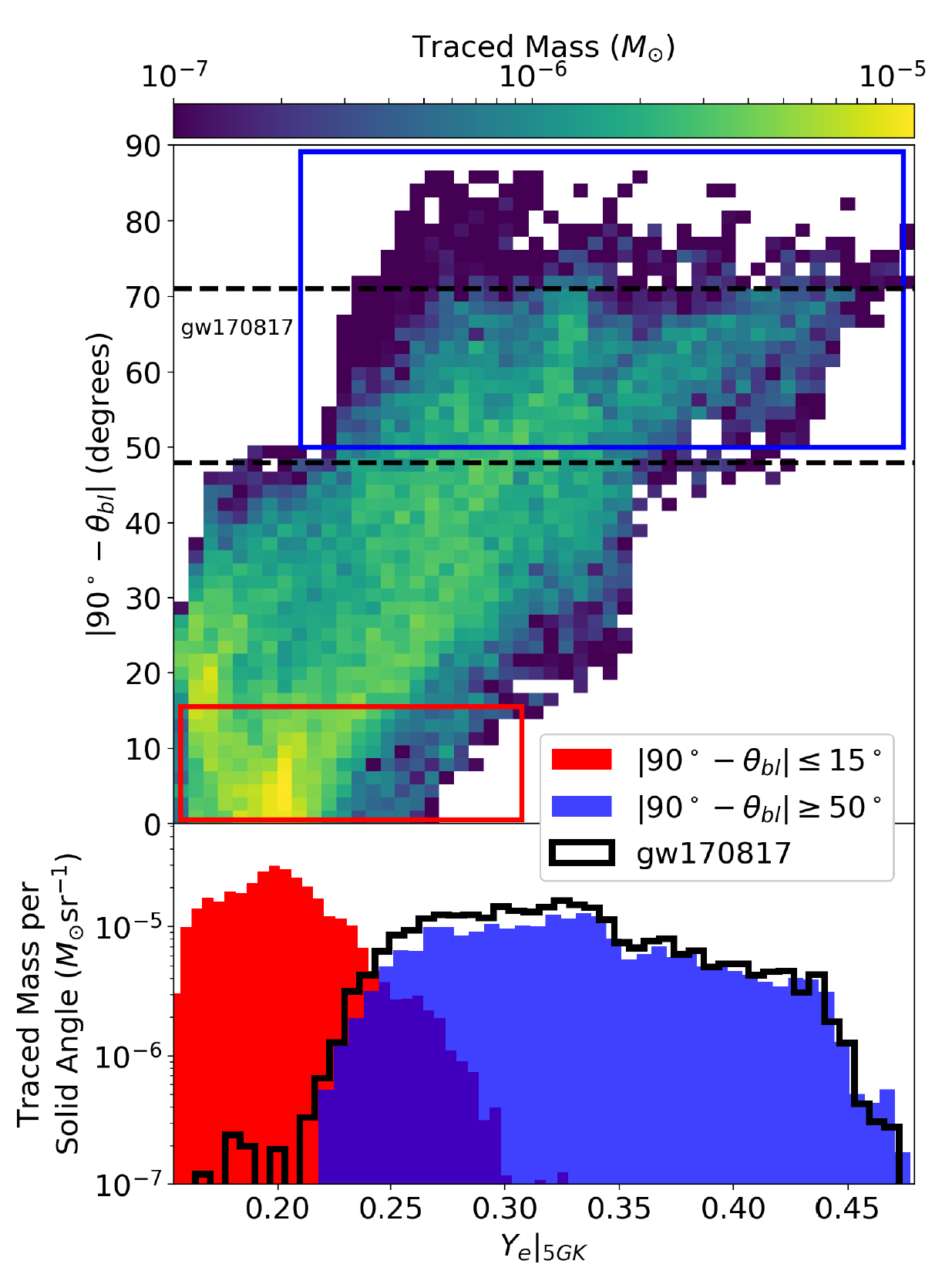Surrounding a black hole

Viewing angle critical for what composition you see

Measured from the mid-plane of the disk

This is consistent with observations of a blue kilonova

Red, full $r$-process material seen near mid-plane

The disk can contain a large amount of material

But exactly how much is gravitationally unbound?

Further... What is the important role of neutrinos?

We need more analysis of these simulations...

Miller et al. submitted (2019)

Neutrinos impact the nucleosynthesis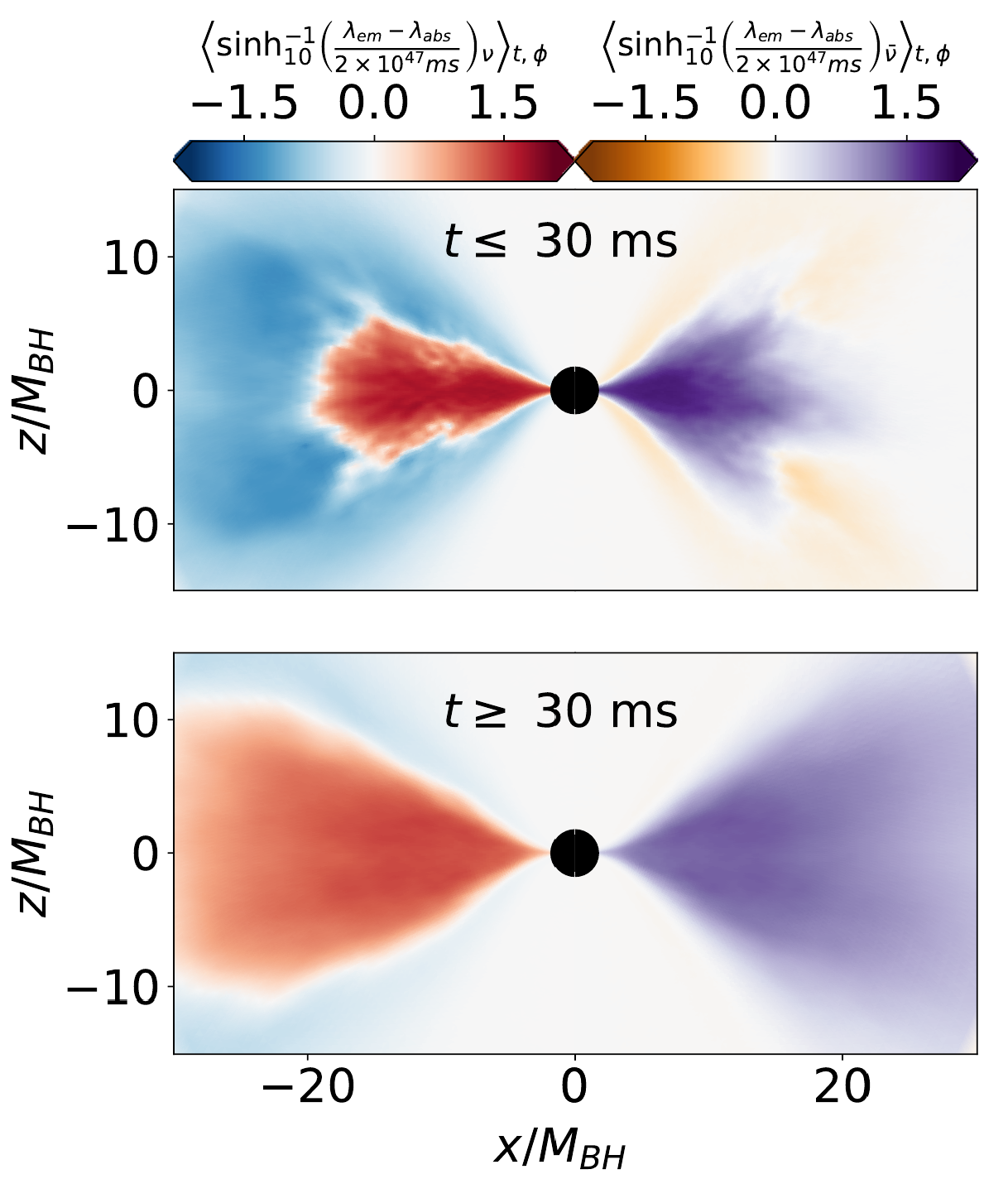Neutrino absorption and emission is considered

Sets the $Y_e$ for the nucleosynthesis from disk ejecta

Miller et al. submitted (2019)

Neutrinos impact the nucleosynthesis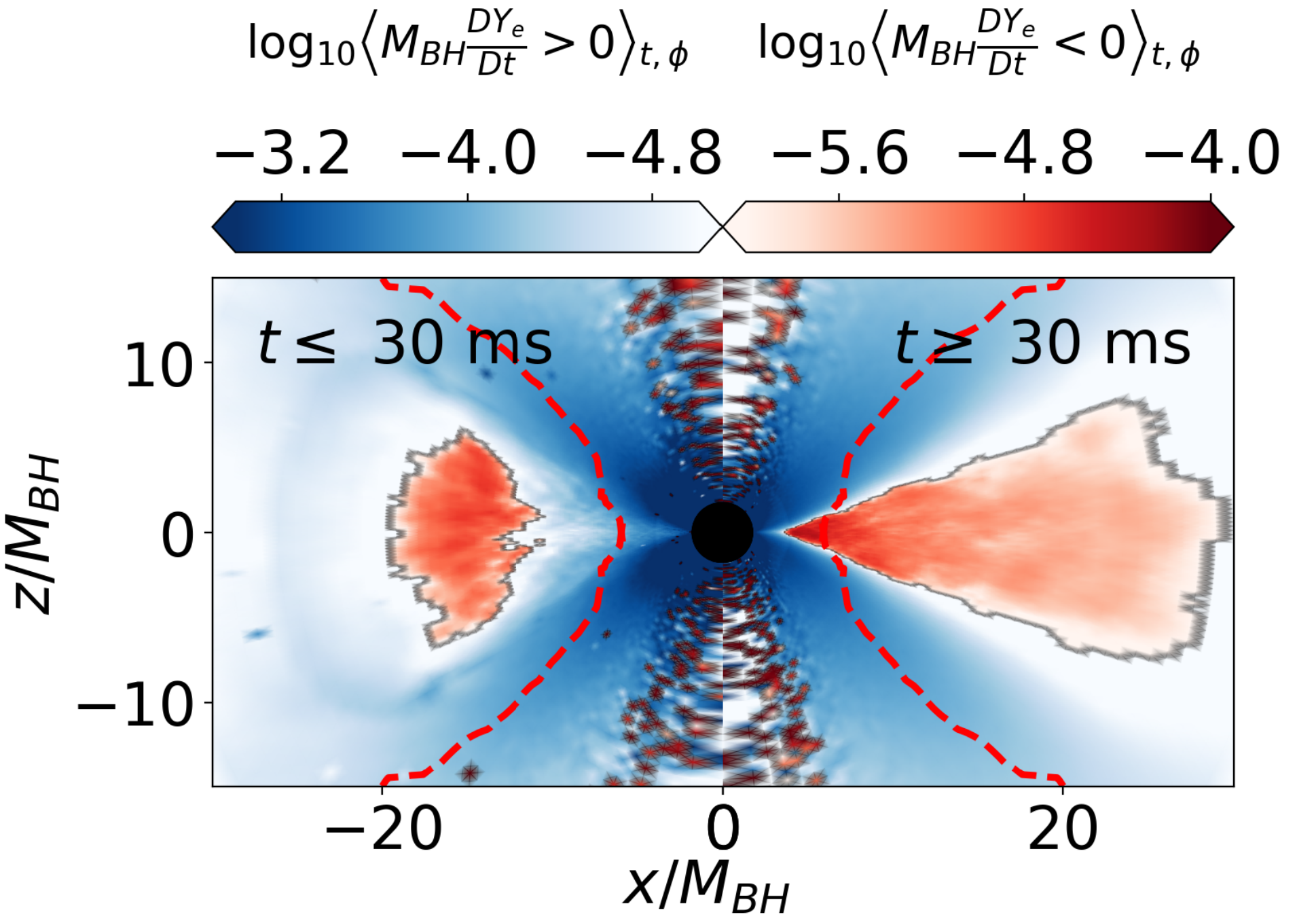Exactly how the ejecta is processed by neutrinos is not fully analyzed yet

Blue region has high $Y_e$; red lower $Y_e$

The transit time away from the disk is crucial

Miller et al. submitted (2019)

Resultant nucleosynthesis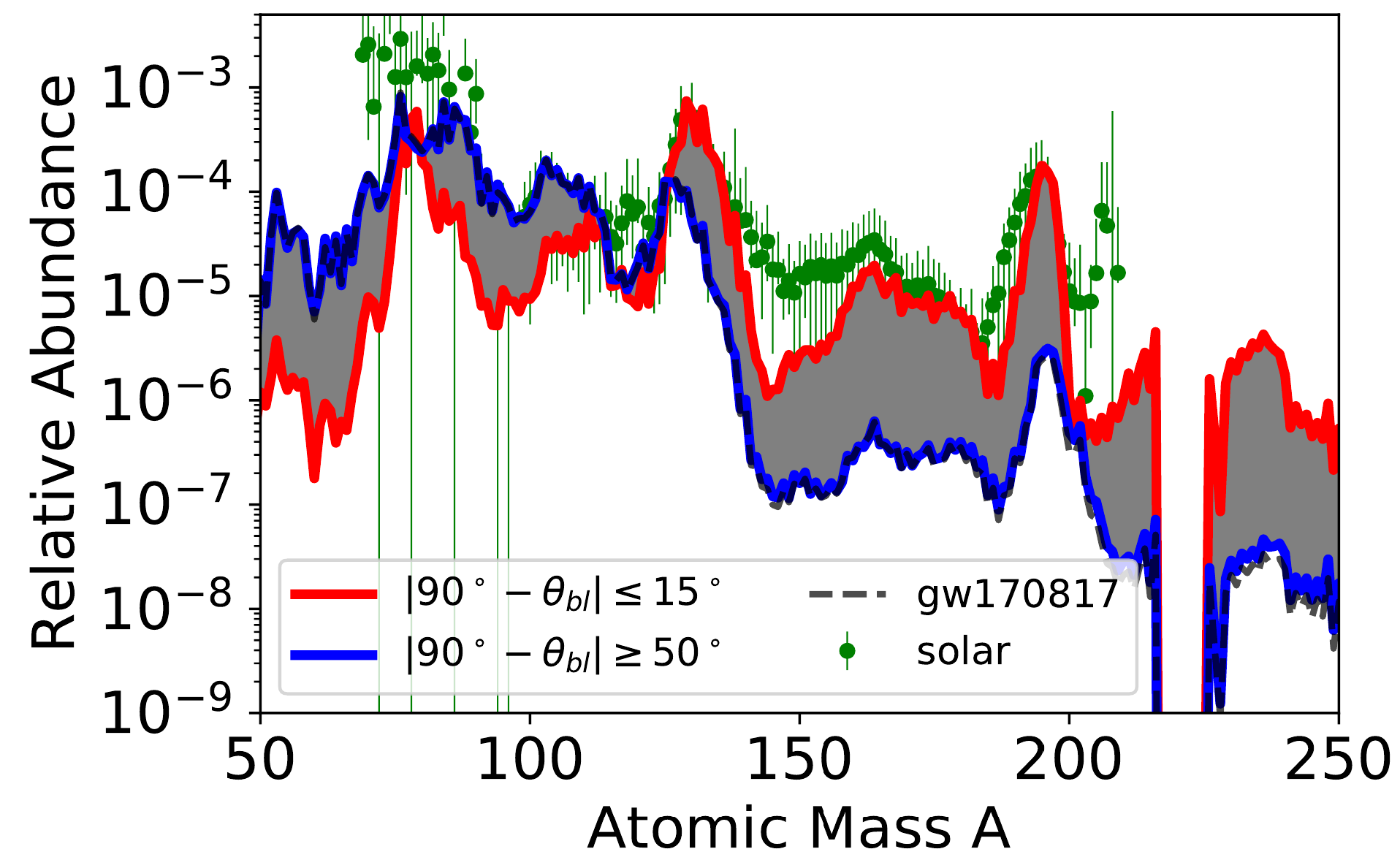Nucleosynthesis simulation with J. Lippuner's SkyNet

Mass weighted trajectories suggest blue kilonova

But it is critical to get the full morphology correct...

Miller et al. submitted (2019)

Merger Morphologies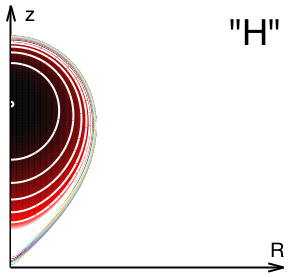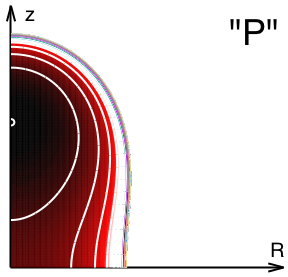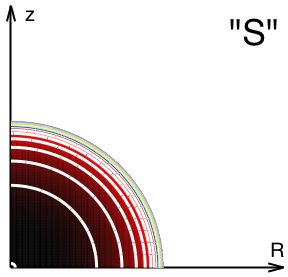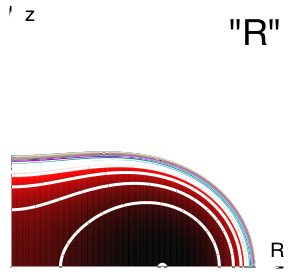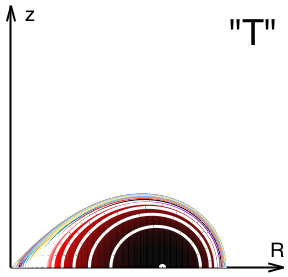Hourglass

Peanut

Sphere

RBC?

Torus

We break down the density into simple constructive components

We study these single components and two component admixtures (1 blue + 1 red)

We want to understand the impact to the lightcurve

Korobkin et al. in prep (2019)

Impact on lightcurves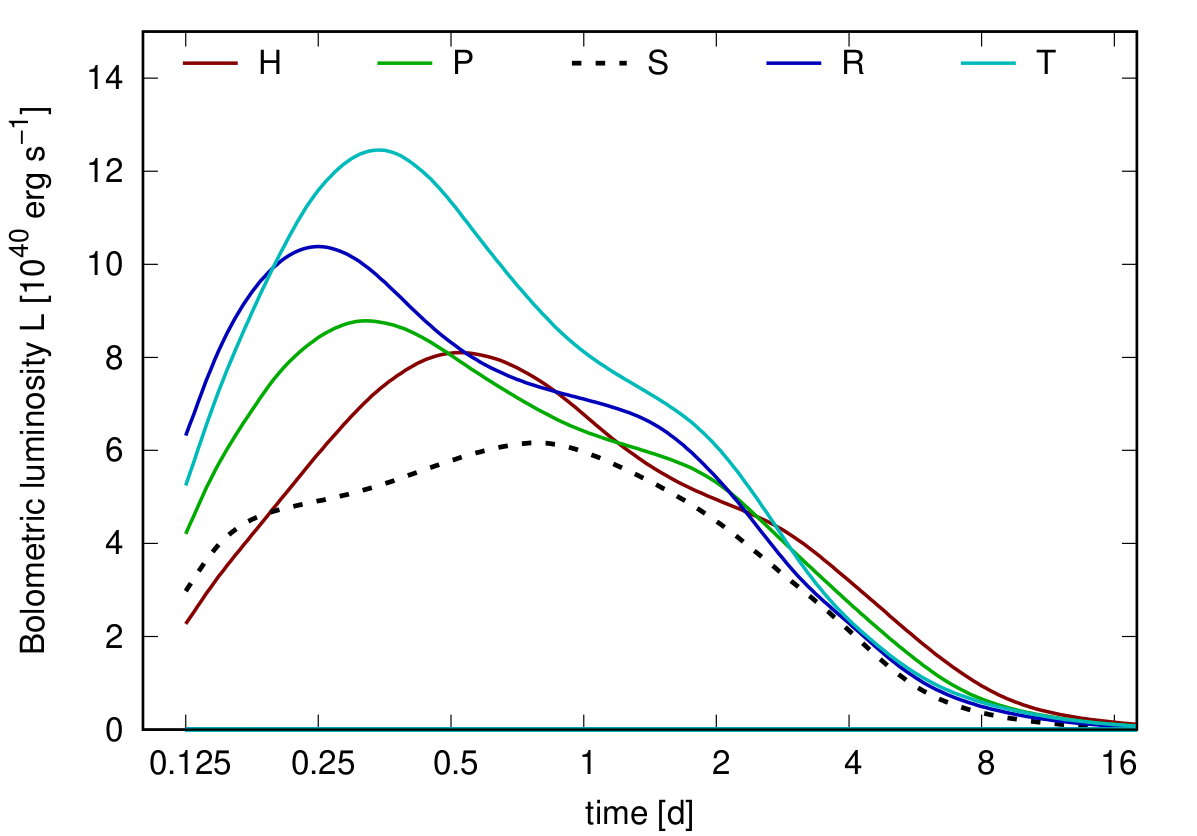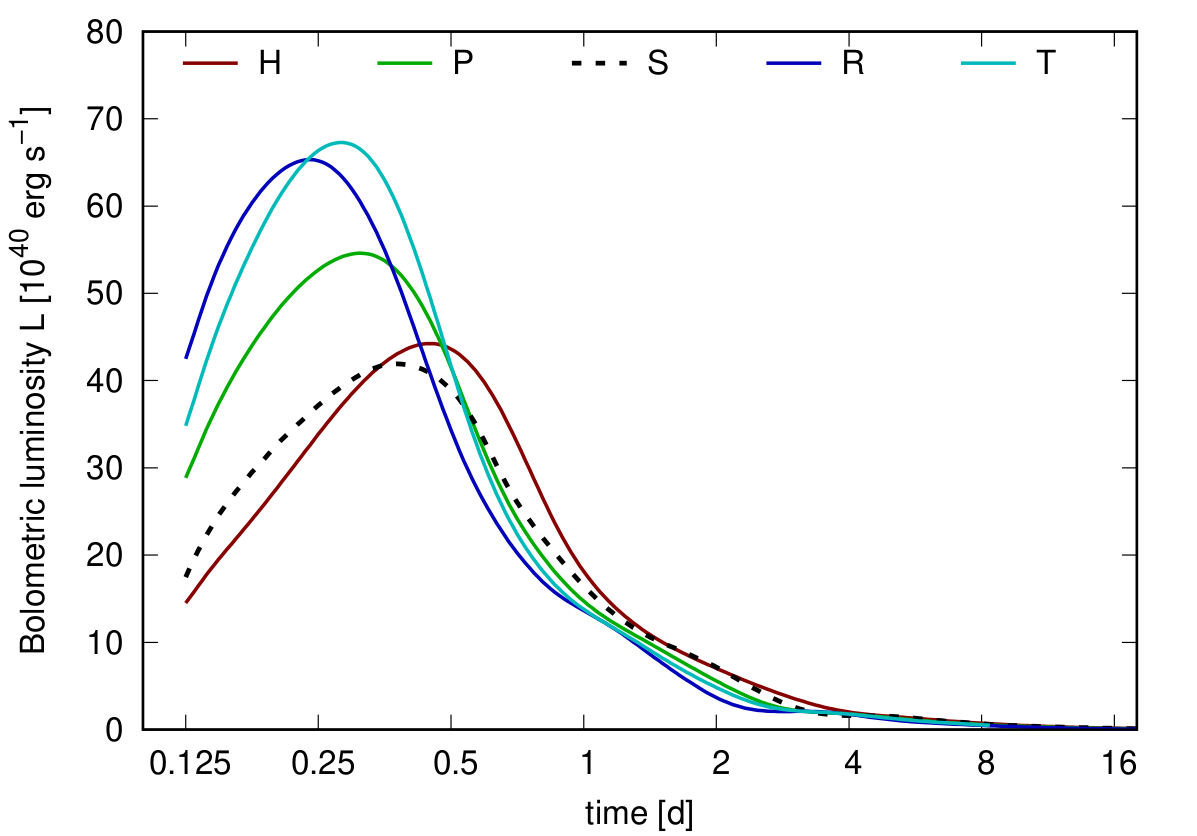Solar-like

$Y_e=0.30$

Brightness of single components is very sensitive to merger morphology

Two components still being analyzed... uncertainty in $M_{\odot}$ is larger than previous studies suggest

Côté et al. ApJ (2018) • Korobkin et al. in prep (2019)

Sensitivity to Nd($Z=60$)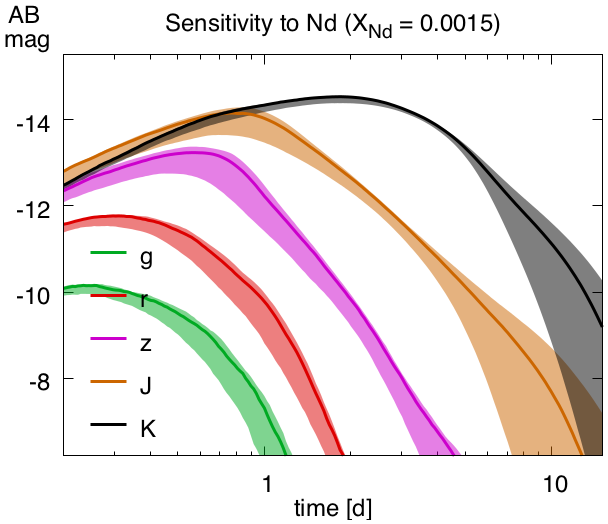Performed sensitivity study of composition of all lanthanides with new LANL line-binned opacities

The presence of even a minor amount of neodymium produces a red kilonova

Uncertainties in opacities further obscure claims of mass ejecta from different components

Fontes et al. submitted (2019) • Even et al. in prep (2019)

Observational Impact of Californium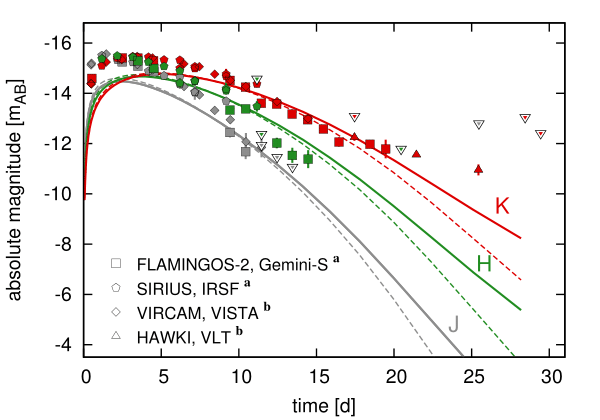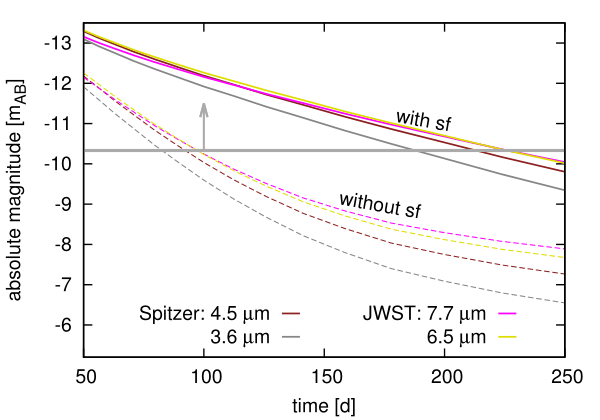Both near- and middle- IR are impacted by the presence of $^{254}$Cf

Late-time epoch brightness can be used as a proxy for actinide nucleosynthesis

Future JWST will be detectable out to 250 days with the presence of $^{254}$Cf

This was the conclusion of previous work with a single component outflow...

Can we ever observe due to merger morphology?...

Y. Zhu et al. ApJL 863 2 (2018) • Korobkin et al. in prep (2019)

Merger $\gamma$-rays

Another possible (yet very difficult) option is to attempt to observe the spectra from transients / remnants

For the $r$-process we should search for signatures of actinides...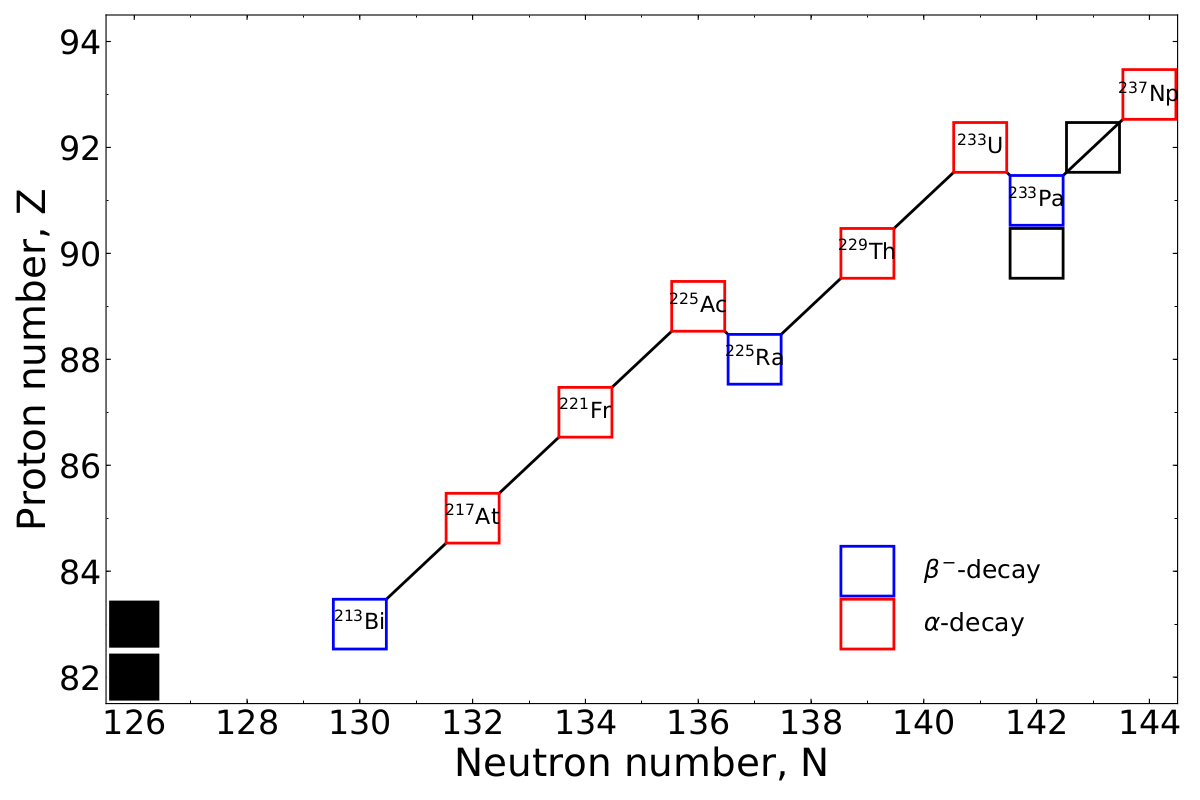This involves following potentially complex decay chains...

Korobkin et al. in prep (2019)

$\gamma$-ray spectrum at 10 kyr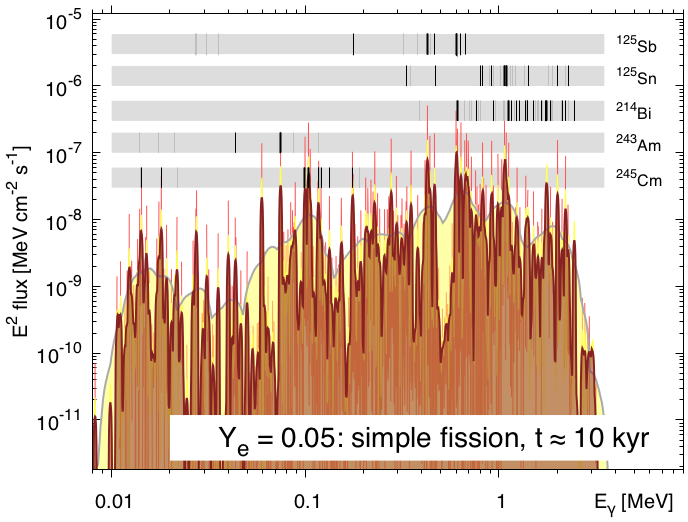Distinct elements do arise

This depends sensitively on observational timescale

Can we do this with future space missions?

Korobkin et al. in prep (2019)

Special thanks to

My collaborators

A. Burrows, J. Dolance, W. Even, C. Fontes, C. Fryer, A. Hungerford, J. Lippuner, J. Miller, W. Misch, O. Korobkin, B. Ryan, T. Sprouse, R. Surman, W. Vestrand, R. Wollaeger

Students Postdocs CTA staff

Summary

Neuron star mergers (NSMs) are a promising site for heavy element synthesis

New insights into neutron star mergers from CTA:

Accretion disksMorphology & compositionLate-time observations

The material from accretion disks may contain the bulk of the ejecta from a merger

Observations depend on angle & morphology & composition

This is important if we want to prove definitively that heavy elements such as the actinides were made in NSMs

Future space missions may be able to detect $\gamma$-rays from long-lived radioactive decay

Results / Data / Papers @ MatthewMumpower.com